HOSTS- Jeremy Burns, Matthew Scott Phillips

TYPE- Theory

DURATION- 34:34

BUMPER MUSIC- "The Sigh" (Matthew Scott Phillips)

ANNOUNCER- Mike Cunliffe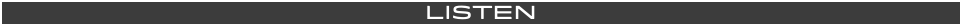Understanding intervals is an essential part of understanding, analyzing and critically listening to music. In this episode, we will discuss the theory behind intervals. Listen and learn the types of intervals and their rolls. Find out some tips how to identify them by number and by quality (major, minor, augmented or diminished). We will distinguish the difference between simple and compound intervals. This episode, like many theory episodes, will have an ear training counter part in the near future!INTERVAL- The distance between two notes, harmonically or melodically. An interval consists of two components: 1) NUMBER and 2) QUALITY.

UNISON- When two instruments play the same pitch, often at the same time. For example, if a pianist and a violinist are both playing A440, then they are said to be playing in UNISON.

PERFECT INTERVAL- Typically, the distance (from the root note) to the notes that land on the 4th, 5th and 8th (unison) scale degrees. These note are the same in both major and minor keys.

MAJOR INTERVAL- Typically, the distance (from the root note) to the notes that land on the 2nd, 3rd, 6th and 7th scale degrees in the MAJOR scale.

MINOR INTERVAL-Typically, the distance (from the root note) to the notes that land on the 2nd, 3rd, 6th and 7th scale degrees in the MINOR scale.

AUGMENTED INTERVAL- When a MAJOR interval is raised by a half step.

DIMINISHED INTERVAL- When a MINOR interval is lowered by a half step.

SIMPLE INTERVAL- An interval that spans the space of 1 octave.

COMPOUND INTERVALS- An interval that spans the space beyond 1 octave.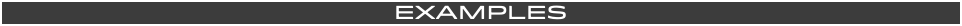We can find the NUMBER of an interval by counting the scale steps from the starting note to the one in question.

The NUMERIC DISTANCE from D to G is 4.  In the highlighted area below, we see eighth notes moving up the D major scale. There are 4 scale degrees

(or scale steps) between the notes: D and G. This means that the interval between these two notes is that of a 4th.We can find the QUALITY of an interval by determining the scale it belongs to. If the highest note, of that interval, is a note that is in the major scale of the lowest note, then that interval is ether

MAJOR or PERFECT.

Below, in the first measure, we see the notes E (on the bottom) and C# (on the top). We see, based on the key signature, that we are in E major. As depicted in the following measure, there are 6 scale  degrees between these two notes. We now know that the NUMBER of this interval is that of a SIXTH. Because the note, C#, is in the E major scale, we know that the interval is MAJOR, in quality.

This interval is a MAJOR SIXTH (M6).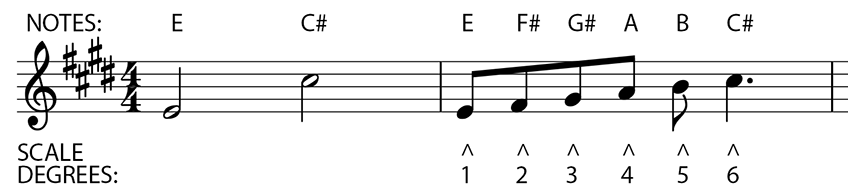Below we see that the intervals of 1 (unison), 4, 5 and 8 are considered to be PERFECT,

in both the MAJOR and MINOR keys.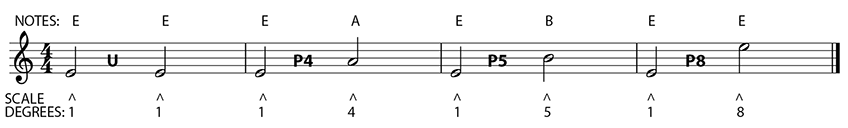If the top note is not in the MAJOR scale of the bottom note, me must determine if it's flat or sharp.

Below, we see E (bottom) and G (top).

We can tell, by the provided key signature, that we are in E major.

The note given (E natural) is a 1/2 step below E# (scale step 3 in the key of E major). Because this scale degree has been flatted,we now know that this interval is that of a

MINOR THRID (m3).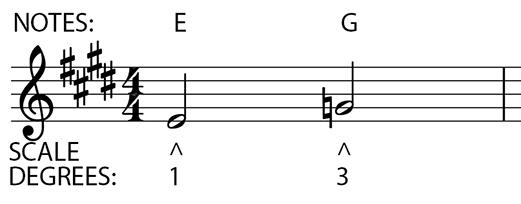If an interval is a 1/2 step below a MINOR INTERVAL,

it is said to be a

DIMINISHED INTERVAL.If an interval is a 1/2 step above a MAJOR INTERVAL,

it is said to be an

AUGMENTED INTERVAL.SIMPLE INTERVALS

Simple intervals span the space of one octave. Below we see all the SIMPLE INTERVALS

that occur from one E note to the next E note.

This distance spans 1 octave.COMPOUND INTERVALS

Compound intervals span the space beyond one octave. Below we see all the

COMPOUND INTERVALS that occur from the E note beyond that initial octave.-Intervals consist of two components: NUMBER AND QUALITY

-When calculating interval distances, start with NUMBER first. Then figure out the QUALITY.

-To simplify, one could determine the NUMERIC value of an interval by counting each notes letter name as a number, as you go up or down the scale, from that note to the one in question.

-The intervals of the 4th, 5th and 8th are all said to be PERFECT in their natural state.

-If the 4th or 5th scale degree is lowered or sharpened, then it is considered to be diminished or augmented, respectively.

-Doubly DIMINISHED and AUGMENTED intervals exist, though they occur in rare circumstances.

- An understanding of the OVERTONE SERIES can help us understand why some intervals are considered to be more CONSONANT (stable or pleasing) or more DISSONANT (jarring and tense)."The Sigh"

(Matthew Scott Phillips)

MUSIC STUDENT 101